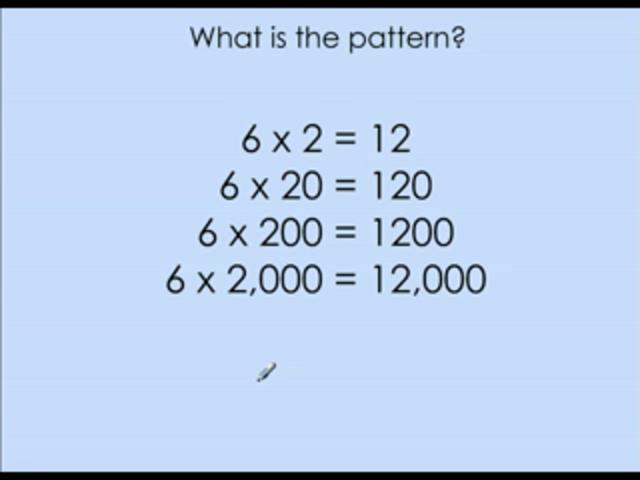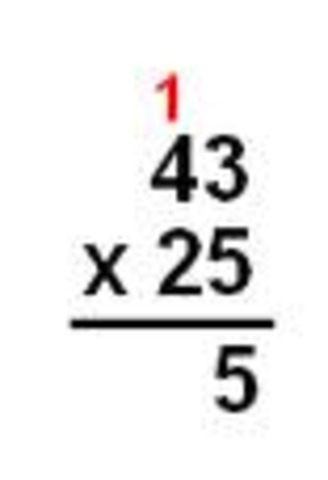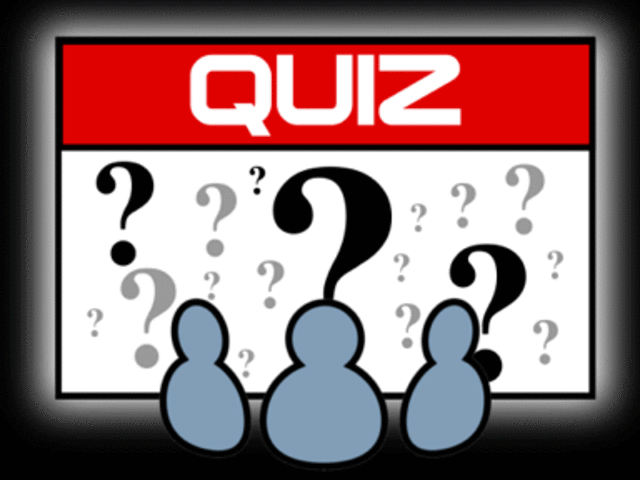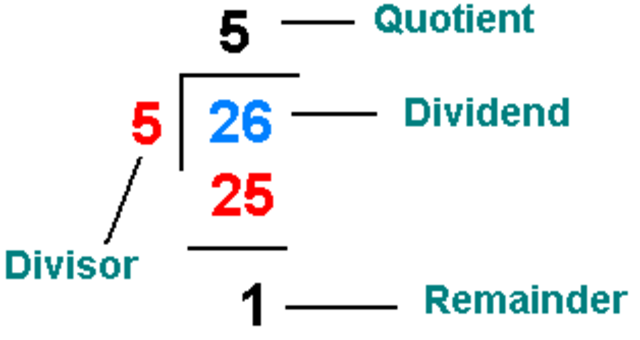# Grade 4: Number Sense and Numeration - Multiplication and Division (February/March 2013)

Timeline created by clagast
• # Lesson 1: Introduction to MultiplicationIn this lesson we will be introduced to the basics of multiplication. In this lesson we will learn how to use the multiplication chart and how multiplication is related to other kinds of math. For example, multiplication is not only repeated addition, but is also the opposite of division.
• # Lesson 2: Estimating ProductsIn this lesson we will be learning how to estimate products. Do you remember how to round to the nearest 10? Great! That means you can estimate. We will learn to solve questions similar to the one below. A basket holds 23 apples. How many apples does 5 baskets hold? We know that the number 23 would round down to 20.
That means we have to solve 5x20
5x20=100 5 baskets hold approximately 100 apples.
• # Lesson 3: Multiplication PatternsIn this lesson we will be learning various multiplication patterns. We will learn how we can use basic multiplication facts to solve for more difficult facts. For example, we will learn to solve questions similar to the one below. 4x6=24
4x60=240
4x600=2400
4x6000=24000
• # Lesson 4: Multiplying 2-digit by 1-digit numbersIn this lesson we will learn how to multiply 2-digit by 1-digit numbers. It is much easier than it looks! We will learn to solve questions similar to the ones below. 23 72 64
x 2 x 6 x 3 Click here to take a sneak peak on how to multiply 2-digit by 1-digit numbers.
• # Lesson 5: QuizQuiz Time! This quiz will be on the following topics:
- basic multiplication facts
- estimating products
- patterns
- multiplication of 2-digit by 1-digit numbers To prepare for the quiz, complete pages 254-255 in the Math Makes Sense textbook. Good Luck!
• # Lesson 6: Dividing Numbers from 1 to 9In this lesson we will learn to divide numbers from 1 to 9. We will learn to divide using arrays and counter manipulatives. This is one of my favorite lessons! For this lesson, please feel free to bring anything from home that you use to help you count. Remember, please bring appropriate counters like buttons, beads and coins.
• # Lesson 7: Long DivisionIn this lesson we will learn how to divide using long division. For many students it takes 2-3 lessons to learn how to divide using long division. Do not worry if you are one of these students. We will spend as much time as we need to become long division pros! In order to prepare for this lesson you can familiarize yourself with the words of the long division process. See how many times you can say divide, multiply, subtract and bring down.
• # Lesson 8: QuizQuiz Time! This quiz will be on the following topics:
- division from 1-9 using arrays and counter manipulatives
- long division In order to prepare for the test complete pages 262-263 in your Math Makes Sense textbook. Good Luck!
• # Lesson 9: Show What You KnowThis lesson will be a review for our upcoming test. During this lesson we will review multiplication facts, multiplication patterns, estimating, multiplication of 2-digit by 1-digit numbers and division. During this lesson there will also be a time set aside for questions and answers. Please come prepared with any questions that you may have so we can make the most of this review session.
• # Lesson 10: Show What You KnowThis lesson will be a continuation of last day. Please come to class with any questions that you may have so we can make the most of this review session.
• # Lesson 11: Unit TestUnit test time! My goodness this unit went by so quickly! This unit test will test you on the following topics:
- multiplication facts 1 to 9
- multiplication patterns
- estimating
- multiplication of 2-digit by 1-digit numbers
- division using arrays
- long division
- word problems Best of luck grade 4's!